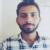true

Take Class 10 Tuition from the Best Tutors

•Affordable fees
•1-1 or Group class
•Flexible Timings
•Verified Tutors

Search in

# Formula of A PManjeet Khajuria
19/10/2022 00

an = a+(n-1)d

Where an=last term

a=first term

d=common difference

n=number of term

Note: last term is denoted by an or l

Sn=n/2[2a+(n-1)d

Sn=sum of all terms

0 Dislike
Follow 1## Other Lessons for You

Example on Probability
Mathematical Statistics. Probability Q1. The probability that a student will pass the final examination in both...Q: The numerator of a fraction is 3 less than the denominator. If 2 is added to both the numerator and the denominator, then the sum of the new and the original fraction is 29/20. Find the...### Looking for Class 10 Tuition ?

Learn from Best Tutors on UrbanPro.

Are you a Tutor or Training Institute?

Join UrbanPro Today to find students near you

X

### Looking for Class 10 Tuition Classes?

The best tutors for Class 10 Tuition Classes are on UrbanPro

• Select the best Tutor
• Book & Attend a Free Demo
• Pay and start Learning### Take Class 10 Tuition with the Best Tutors

The best Tutors for Class 10 Tuition Classes are on UrbanPro